# Loops with unknown nesting depth

We often encounter nested loops, like

```for (i = 0; i < n; i++) {
for (j = 0; j < m; j++) {
doSomething(i, j);
}
}
```

This can be nested to a few more levels without too much pain, as long as we observe that the number of iterations for each level need to be multiplied to get the number of iterations for the whole thing and that total numbers of iterations beyond a few billions (, German: Milliarden, Russian Миллиарди) become unreasonable no matter how fast the `doSomethings(...)` is. Just looking at this example program

```public class Modular {
public static void main(String[] args) {
long n = Long.parseLong(args);
long t = System.currentTimeMillis();
long m = Long.parseLong(args);
System.out.println("n=" + n + " t=" + t + " m=" + m);
long prod = 1;
long sum  = 0;
for (long i = 0; i < n; i++) {
long j = i % m;
sum += j;
sum %= m;
prod *= (j*j+1) % m;
prod %= m;
}
System.out.println("sum=" + sum + " prod=" + prod + " dt=" + (System.currentTimeMillis() - t));
}
}
```

which measures it net run time and runs 0 msec for 1000 iterations and almost three minutes for 10 billions (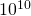):

```> java Modular 1000 1001 # 1'000
--> sum=1 prod=442 dt=0
> java Modular 10000 1001 # 10'000
--> sum=55 prod=520 dt=1
> java Modular 100000 1001 # 100'000
--> sum=45 prod=299 dt=7
> java Modular 1000000 1001 # 1'000'000
--> sum=0 prod=806 dt=36
> java Modular 10000000 1001 # 10'000'000
--> sum=45 prod=299 dt=344
> java Modular 100000000 1001 # 100'000'000
--> sum=946 prod=949 dt=3314
> java Modular 1000000000 1001 # 1'000'000'000
--> sum=1 prod=442 dt=34439
> java Modular 10000000000 1001 # 10'000'000'000
--> sum=55 prod=520 dt=332346
```

As soon as we do I/O, network access, database access or simply a bit more serious calculation, this becomes of course easily unbearably slow. But today it is cool to deal with big data and to at least call what we are doing big data, even though conventional processing on a laptop can do it in a few seconds or minutes... And there are of course ways to process way more iterations than this, but it becomes worth thinking about the system architecture, the hardware, parallel processing and of course algorithms and software stacks. But here we are in the "normal world", which can be a "normal subuniverse" of something really big, so running on one CPU and using a normal language like Perl, Java, Ruby, Scala, Clojure, F# or C.

Now sometimes we encounter situations where we want to nest loops, but the depth is unknown, something like

```for (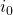= 0;<;++) {
for (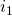= 0;<;++) {for (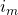= 0;<;++) {
dosomething();
}}
}
```

Now our friends from the functional world help us to understand what a loop is, because in some of these more functional languages the classical C-Style loop is either missing or at least not recommended as the everyday tool. Instead we view the set of values we iterate about as a collection and iterate through every element of the collection. This can be a bad thing, because instantiating such big collections can be a show stopper, but we don't. Out of the many features of collections we just pick the iterability, which can very well be accomplished by lazy collections. In Java we have the `Iterable`, `Iterator`, `Spliterator` and the `Stream` interfaces to express such potentially lazy collections that are just used for iterating.

So we could think of a library that provides us with support for ordinary loops, so we could write something like this:

```Iterable range = new LoopRangeExcludeUpper<>(0, n);
for (Integer i : range) {
doSomething(i);
}
```

or even better, if we assume 0 as a lower limit is the default anyway:

```Iterable range = new LoopRangeExcludeUpper<>(n);
for (Integer i : range) {
doSomething(i);
}
```

with the ugliness of boxing and unboxing in terms of runtime overhead, memory overhead, and additional complexity for development. In Scala, Ruby or Clojure the equivalent solution would be elegant and useful and the way to go...
I would assume, that a library who does something like `LoopRangeExcludeUpper` in the code example should easily be available for Java, maybe even in the standard library, or in some common public maven repository...

Now the issue of loops with unknown nesting depth can easily be addressed by writing or downloading a class like `NestedLoopRange`, which might have a constructor of the form `NestedLoopRange(int ... ni)` or `NestedLoopRange(List li)` or something with collections that are more efficient with primitives, for example from Apache Commons. Consider using long instead of int, which will break some compatibility with Java-collections. This should not hurt too much here and it is a good thing to reconsider the 31-bit size field of Java collections as an obstacle for future development and to address how collections can grow larger than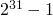elements, but that is just a side issue here. We broke this limit with the example iterating over 10'000'000'000 values for i already and it took only a few minutes. Of course it was just an abstract way of dealing with a lazy collection without the Java interfaces involved.

So, the code could just look like this:

```Iterable range = new NestedLoopRange(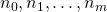);
for (Tuple t : range) {
doSomething(t);
}
```

Btw, it is not too hard to write it in the classical way either:

```        long[] n = new long[] {};
int m1 = n.length;
int m  = m1-1; // just to have the math-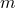matched...
long[] t = new long[m1];
for (int j = 0; j < m1; j++) {
t[j] = 0L;
}
boolean done = false;
for (int j = 0; j < m1; j++) {
if (n[j] <= 0) {
done = true;
break;
}
}
while (! done) {
doSomething(t);
done = true;
for (int j = 0; j < m1; j++) {
t[j]++;
if (t[j] < n[j]) {
done = false;
break;
}
t[j] = 0;
}
}
```

I have written this kind of loop several times in my life in different languages. The first time was on C64-basic when I was still in school and the last one was written in Java and shaped into a library, where appropriate collection interfaces were implemented, which remained in the project or the organization, where it had been done, but it could easily be written again, maybe in Scala, Clojure or Ruby, if it is not already there. It might even be interesting to explore, how to write it in C in a way that can be used as easily as such a library in Java or Scala. If there is interest, please let me know in the comments section, I might come back to this issue in the future...

In C it is actually quite possible to write a generic solution. I see an API like this might work:

```struct nested_iteration {
/* implementation detail */
};

void init_nested_iteration(struct nested_iteration ni, size_t m1, long *n);
void dispose_nested_iteration(struct nested_iteration ni);
int nested_iteration_done(struct nested_iteration ni); // returns 0=false or 1=true
void nested_iteration_next(struct nested_iteration ni);
```

and it would be called like this:

```struct nested_iteration ni;
int n[] = {};
for (init_nested_iteration(ni,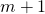, n);
! nested_iteration_done(ni);
nested_iteration_next(ni)) {
...
}
```

So I guess, it is doable and reasonably easy to program and to use, but of course not quite as elegant as in Java 8, Clojure or Scala.
I would like to leave this as a rough idea and maybe come back with concrete examples and implementations in the future.